Zhang, Y., Wang, L., Wu, Y., Chen, Y., Ma, H., Zhou, H., Zhu, Z., Liu, S., and Zhou, J. (2019). "Pyrolysis characteristics, kinetics, and its product characteristics of grape stem," BioRes. 14(4), 7901-7919.

#### Abstract

Grape stem is a kind of agricultural and forestry waste. A fundamental understanding of grape stem pyrolysis behavior and kinetics is essential for its efficient thermochemical conversion. Thermogravimetric infrared spectroscopy and pyrolysis gas chromatography-mass spectrometry, combined with two model-free integral methods: Flynn-Wall-Ozawa (FWO) and Kissinger-Akahira-Sunose (KAS) were used to investigate the weight loss behavior, the distribution and content of rapid pyrolysis products, the release law of small molecule pyrolysis gases, and the pyrolysis activation energy during pyrolysis. The results showed that the main pyrolysis reaction temperature ranged from 240 °C to 690 °C. The pyrolysis reaction of grape stems at 200 °C to 700 °C was divided into three stages: 0.15 < α < 0.35, 0.35 < α < 0.65, and 0.65 < α < 0.75, which corresponded to the main pyrolysis stages of hemicellulose, cellulose, and lignin, respectively. The products of rapid pyrolysis at 290 °C were mainly composed of acids and sugars, while the products at 355 °C were mainly phenolics. This study aims to provide a theoretical reference for the pyrolysis gasification test of grape stem.

Pyrolysis Characteristics, Kinetics, and Its Product Characteristics of Grape Stem

Yimeng Zhang,# Liangcai Wang,# Yishuang Wu, Yu Chen, Huanhuan Ma, Hao Zhou, Zhengxiang Zhu, Shasha,Liu, and Jianbin Zhou *

Grape stem is a kind of agricultural and forestry waste. A fundamental understanding of grape stem pyrolysis behavior and kinetics is essential for its efficient thermochemical conversion. Thermogravimetric infrared spectroscopy and pyrolysis gas chromatography-mass spectrometry, combined with two model-free integral methods: Flynn-Wall-Ozawa (FWO) and Kissinger-Akahira-Sunose (KAS) were used to investigate the weight loss behavior, the distribution and content of rapid pyrolysis products, the release law of small molecule pyrolysis gases, and the pyrolysis activation energy during pyrolysis. The results showed that the main pyrolysis reaction temperature ranged from 240 °C to 690 °C. The pyrolysis reaction of grape stems at 200 °C to 700 °C was divided into three stages: 0.15 < α < 0.35, 0.35 < α < 0.65, and 0.65 < α < 0.75, which corresponded to the main pyrolysis stages of hemicellulose, cellulose, and lignin, respectively. The products of rapid pyrolysis at 290 °C were mainly composed of acids and sugars, while the products at 355 °C were mainly phenolics. This study aims to provide a theoretical reference for the pyrolysis gasification test of grape stem.

Keywords: Grape stem; Pyrolysis; Kinetics; TGA-FITR; PY-GCMS

Contact information: College of Materials Science and Engineering, Nanjing Forestry University, Nanjing 210037, China; #These authors contributed equally to this work;

*Corresponding author: 13705178820@163.com

INTRODUCTION

The decline in the amount of fossil fuels, increase in environmental pollution, and increased demand for safe fuels, chemicals, and energy have contributed to increased interest in renewable energy (Lopez-Velazquez et al. 2013). Biomass is one of the alternatives to fossil fuels as a renewable and sustainable resource (Kim et al. 2013). The use of biomass not only can reduce the reliance on limited fossil fuels, but also it could have a positive impact on the environment, such as the reduction in the release of carbon dioxide and sulfur oxides (Siti et al. 2012; Mythili et al. 2013).

Grape stems are woody vines that grow from the trunk of the grape vine for many years. To ensure the yield and quality of grapes, people need to trim them regularly during the growth process of grapes. With the scaling-up of grape planting, the number of plucked grape stems are considerably higher every year. If not utilized properly, it can cause environmental pollution and even harm the ecological balance (Wang and Frank 2005). Pyrolysis is one of the most common methods of converting agricultural and forestry waste into valuable fuel chemicals, and an important sub-step of gasification technology yielded producer gas for electricity supply (Ma et al. 2012; Narobe et al. 2014). The chemical components in biomass are broken down into low molecular weight gases (volatiles), liquids (tar), and solid char after pyrolysis (Damartzis et al. 2011; Geng et al. 2017). Combustible gaseous and liquid products can be used as fuel due to their high calorific value. The biochar produced can be used to prepare activated carbon, mechanical carbon, and carbon-based fertilizers. Therefore, research on the pyrolysis process of agricultural and forestry waste will help to better explain its pyrolysis mechanism and improve its performance as biofuels, chemical products, and biological materials (Lopez-Velazquez et al. 2013). However, the properties of biomass significantly affect heat transfer and reaction rates, resulting in large variations in optimal operating conditions (Hani et al. 2012; Lopez-Velazquez et al. 2013).

Thermogravimetric analysis (TGA), coupled with Fourier transform infrared spectrometry (FTIR), is a good means by which to study not only the mass-loss characteristics and kinetics parameters of the thermal decomposition process, but also identify the volatile components generated in real-time (Ma et al. 2015). Thermal analysis kinetics is an important approach to study the mechanisms of the thermochemical conversion of biomass. Non-isothermal kinetics can be classified into model-free and model-fitting categories. Both methods have their benefits. They are complementary rather than competitive (Lah et al. 2013). Recently, the model-free method, also called the iso-conversional method, has been the most commonly used method in the kinetics study of biomass pyrolysis process (Agrawal and Chakraborty 2013; Zhao et al. 2013). The model-free methods can be split in two categories: differential and integral (Lah et al. 2013). Due to employing the instantaneous rate value, the differential iso-conversional method is sensitive to experimental noise, which makes the numerical value unstable. However, this phenomenon will be effectively avoided by using integral method, especially in TGA experimentation. The Flynn-Wall-Ozawa (FWO) integral method and Kissinger-Akahira-Sunose (KAS) integral method using different approximations are two typical methods (Flynn and Wall 1966; Ma et al. 2015). Thus, they were used to estimate the activation energy of grape stem pyrolysis in this paper.

Extensive research has been performed on grape stems, focusing on composting (Li and Zhang 2000; Wang and Frank 2005), biogasification (Hagos et al. 2017), extraction of functional substances (Anastasiadi et al. 2012; Tosi et al. 2013), etc. Unfortunately, these treatments have some drawbacks. For example, the grape stems cannot be directly used for composting treatment due to the high C/N ratio and big granularity, which means it needs pretreatment. In other words, one cannot totally use the grape stem resources as such (Wang and Frank 2005). In another example, even though biogasification treatment has made great breakthroughs in technology, there is still a low level of available equipment for manufacturing. People are still in the research stage of fertilizing utilization of biogas slurry and biogas residue. Additionally, the processing of grape stem biogasification raw materials is also difficult (Anastasiadi et al. 2012; Hagos et al. 2017). Lastly, when extracting functional substances from grape stems, modeling the extraction process could not be finished easily due to the varieties of grape stem. However, the fixed carbon, C and N of the grape stem are close to those of rice husk, but the ash content is lower than that of the rice husk, and the volatile content is higher than that of the rice husk. Therefore, the grape stem has more pyrolysis potential than rice husks (Chen et al. 2014). With the combined use of TGA and FTIR, the volatile components of biomass pyrolysis process, such as poplar wood and pine wood sawdust, were identified. The main components were some small molecular mass gases (CO, CO2, H2O, CH4) and various kinds of organic compounds. The identification is on the basis of the characteristic absorbances of the functional groups in the evolved gases (Gu et al. 2013; Gao et al. 2013).

Although many studies have been conducted on biomass pyrolysis, few studies have combined the two approaches of TGA-FTIR and Py-GC/MS. This study may be the first attempt to focus on the characteristics, kinetics, and its product characteristics of the grape stems pyrolysis process.

The key objective of this study was to investigate the pyrolysis characteristics, kinetics, and its product characteristics of grape stem using TGA-FTIR and PY-GCMS analysis. Then, two model-free integral method (FWO and KAS) were used to calculate the activation energy describing the thermal devolatilization mechanism of the grape stem pyrolysis processes with different conversion rate (α), using multi-heating rate method (heating rates of 10, 20, 30, 40, and 50 °C/min). This study would be helpful in effective design and operation of pyrolysis gasification test by grape stem.

EXPERIMENTAL

Materials

The grape stem was obtained from the wine production area of Yinchuan (Ningxia, China), and was ground to a fine powder. The powder was passed through to 40-to 60-mesh sieves, allowing particles of sizes from 250 to 380 um to pass, which is suitable for component, TGA-FTIR, and PY-GCMS analyses. The proximate analysis of the grape stem was performed according to the Chinese National Standards GB/T 28731-2012 (proximate analysis of solid biofuels). The ultimate analysis was carried out following the CHNS/O model using an elemental analyzer (Vario macro cube, Elementary, Karlsruhe, Germany), and oxygen was estimated by the difference: O(%) = 100% – C(%) – H(%) – N(%) – S(%) – Ash(%). The results are listed in Table 1. The grape stem powder was dried for 8 h at 105 °C before TGA-FTIR and PY-GCMS analysis was conducted.

Table 1. Ultimate and Proximate Analysis of Grape Stem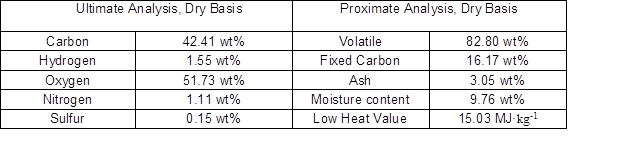Methods

TGA-FTIR analyses

The TGA-FTIR test set up consisted of a thermogravimetric analyzer (TGA STA8000; PerkinElmer, Waltham, MA, USA) and a Fourier transform infrared spectrometry (Frontier; PerkinElmer, Waltham, MA, USA) apparatus. Approximately 10 mg of gape stem sample was used for each test. The temperature was raised from room temperature to 850 °C under heating rates of 10, 20, 30, 40 and 50 °C/min. The flow rate of the carrier gas (high purity nitrogen) was 50 mL/min. The resolution and spectral region of the FTIR were 4 cm-1 and 4000 to 550 cm-1, respectively, and the spectrum scan was conducted with 8-second intervals.

Kinetics Model Analysis

Non-isothermal kinetic model

The non-isothermal reaction rate Arrhenius equation (Eq. 2) is shown as follows,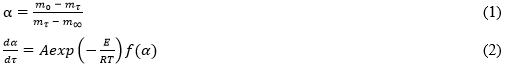where α represents the mass loss number, mrepresents the initial mass (g), mτ represents the mass (g) at the certain time τ (s), m represents the final mass (g) (the mass of the sample cannot be finally reacted after completion of the reaction), A represents the frequency factor (S-1), represents the activation energy (J/mol), T represents the Kelvin temperature, Rrepresents the constant of the molar gas, and f(α) is the reaction mechanism equation. The mass loss α is defined by Eq. 3.

The heating rate β is defined by β = dT/dt, which was obtained by Eq. 2: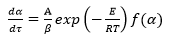(3)

After integrating Eq. 3, one can obtain: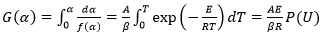(4)

In Eq. 4, G(α) is an integral form of f(α)P(U) is an approximation, and U is defined as U = E/R.

Model-free integral model

To obtain the activation energy E, the grape stems were studied by two integral methods of inorganic function: FWO and KAS, which were expressed by Eqs. 5 and 6, respectively: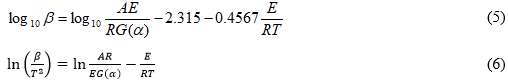The activation energy E can be obtained from a linear relationship between log10β and 1/T and ln(β/T2) and 1/T, for each conversion rate (α), where the slopes is -0.4567E/R and E/R, respectively. Generally, three or more heating rates (10, 20, 30, 40 and 50 °C/min in this study) should be used to obtain reliable values of the activation energy.

PY-GCMS analysis

Approximately 0.5 mg of grape stem powder was weighed into a quartz tube. The initial temperature of pyrolysis was kept at 50 °C, and the final temperature was set to 290, and 355 °C. The heating rate was 20 °C/ms, and the final temperature was maintained for 15 s. Analysis of volatiles was performed using GC-MS (Clarus SQ8; PerkinElmer, Waltham, MA, USA) combined techniques, and the temperature of the lines and syringes were maintained at 300 °C. The chromatographic column used was Elite-5 ms (PerkinElmer, Waltham, MA, USA) (0.25 μm × 0.25 μm × 30 m) and the maximum operating temperature was 350 °C. The carrier gas was He with a purity of 99.999%. The flow rate was maintained at 1.0 mL/min, and the split ratio was 50:1. The column oven was heated from 40 °C (for 2 min) to 295 °C (for 5 min) at the heating speed of 8 °C/min. The mass spectrometer was operated at 70 eV in EI mode. The mass-to-charge ratio was set to 35 to approximately 550 and the scanning rate was 0.002 S-1. The chromatographic peaks were identified by the NIST library and related literature (Brebu et al. 2013).

RESULTS AND DISCUSSION

Analysis of Pyrolysis Process of Grape Stem

Figure 1 shows the TG and differential thermogravimetric (DTG) curves. The TG and DTG curves suggest that the pyrolysis process can be divided into three stages: dry dewatering stage, pyrolysis stage, and pyrolysis-carbonization stage (Chen et al. 2014). In the removal of moisture stage (45 °C to 130 °C), the weight loss rate was approximately 2.6% from the TG curve, and the DTG line had a small concave weight loss peak. The pyrolysis stage (130 °C to 400 °C) is mainly pyrolysis reactions of cellulose and hemicelluloses (Ma et al. 2015; Chen et al.2014). From 130 °C to 210 °C, the thermogravimetric and DTG curves were relatively flat, with little change, and the weight loss was approximately 1.2%. A slow depolymerization process of the grape stem occurred at this stage. Therefore, when drying the raw material, the drying temperature should not be based on the temperature before pyrolysis. The drying temperature should be lower than 130 °C. The temperature range 210 °C to 400 °C was the main pyrolysis reaction weight loss progress of cellulose and hemicellulose, with the sample rapidly pyrolyzing and quickly losing weight, and the weight loss rate reached 58%. The DTG curve showed that a small peak appeared at 295 °C. Because hemicellulose is less stable than lignin and cellulose, the hemicellulose was mainly decomposed in this part; a large peak appeared at 342 °C. In this part, the cellulose mainly underwent a cracking reaction, and the lignin began to crack in the second stage (Ajay et al. 2008). In the third stage, the pyrolysis carbonization stage (400 °C to 800 °C), the lignin was decomposed, producing charcoal and a small amount of ash. The weight loss was approximately 15%, and the carbon obtained by the final pyrolysis was approximately 22.6%.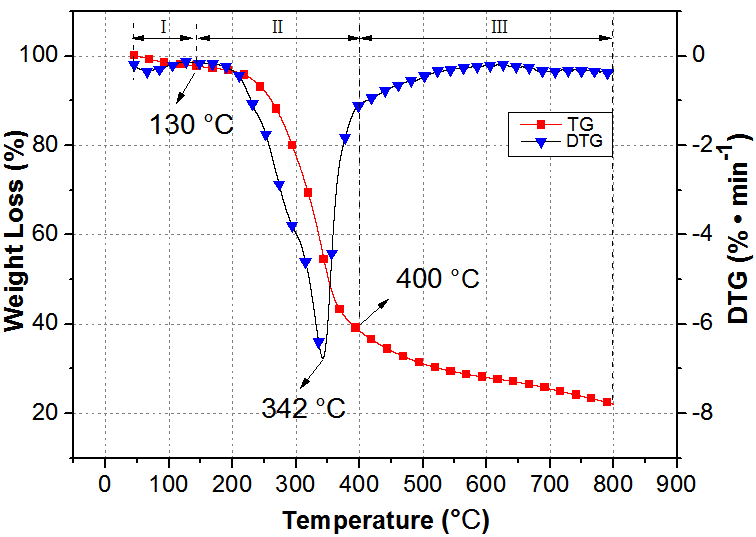Fig. 1. TG and DTG curves of grape stem with rate of 10 °C/min

Effect of Heating Rate on Pyrolysis Characteristics of Grape Stem

Figure 2 shows that the effect of heating rate on the pyrolysis characteristics of grape stems was relatively complicated. As the heating rate increased, the time for the grape stem sample to reach the temperature of pyrolysis was decreased, which was beneficial for rapid pyrolysis (Chen et al. 2014). The true pyrolysis reaction zone was at 240 °C to 690 °C. In this region, with increased heating rate, the thermogravimetric curves moves toward the high temperature region, and the release of volatile components per unit temperature decreases (Kumar et al. 2008; Vyazovkin et al. 2011; Lopez-Gonzalez et al. 2013; Chen et al. 2014). At less than 240 °C and greater than 690 °C many factors, such as moisture and carbonization, affected the pyrolysis progress.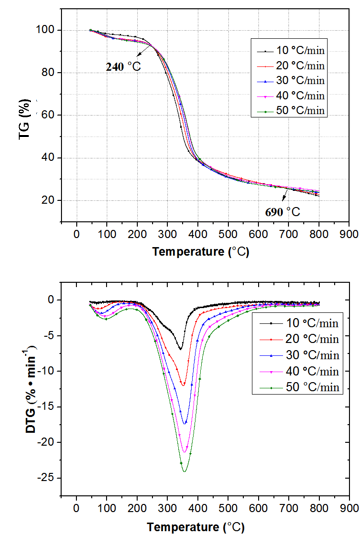Fig. 2. TG and DTG curves of grape stem with five different heating rates

Kinetic Analysis of Grape Stem Pyrolysis

Figure 3 shows the Arrhenius plots based on two model-free methods, namely the FWO and KAS methods, respectively, and the activation energy was calculated at various conversion rates (0.15 to 0.75, with intervals of 0.05 min).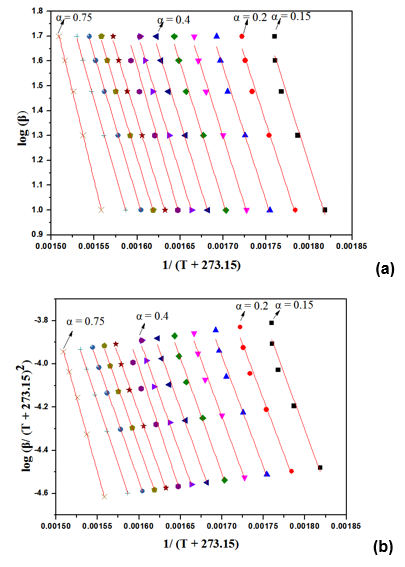Fig. 3. Arrhenius plots of FWO (a), and KAS (b) methods for the conversion rates of 0.15 to 0.75 of grape stem

In Fig. 3(a), the FWO method was used. When the conversion rate was 0.15 to 0.45, the coefficient of determination R2 was 0.98 or more. When the conversion rate was 0.5, Rbecame 0.84; and when the conversion rate was 0.55 to 0.75, R2 was 0.99 or more. In Fig. 3(b), the KAS method was used. The coefficient of determination R2 was 0.98 or more when the conversion rate was 0.15 to 0.4. When the conversion rate was 0.45, R2 was 0.992; when the conversion rate was 0.5, the obtained R2 was 0.83, and when the conversion rate was 0.55 to 0.75, R2 became 0.996 or more. The high similarity and high fitting of the two methods revealed that the activation energy was reliable and the obtained accuracy was relatively high by calculation (Vamvuka et al. 2003).

The distribution of the activation energy is presented in Fig. 4. Within the temperature range of 200 °C to 700 °C, the conversion rate was between 0.15 and 0.75. The activation energy fluctuated up and down with the increase of the conversion rate, which indicated that the grape stem had a complicated and regular chemical reaction during the pyrolysis process. Secondly, Fig. 3 shows the FWO and the KAS methods, where the curves of activation energy and conversion rates displayed similar shapes and small deviations (except for individual points, the deviation was approximately 3%, and the small deviation was caused by the approximation of the algorithm). The two model-free methods verified the accuracy and reliability of the activation energy values again and the measured activation energy was basically the same.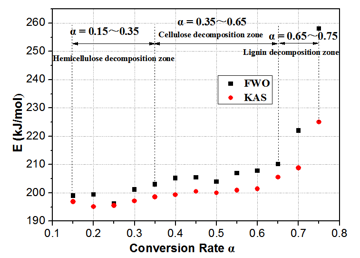Fig. 4. Activation energy change curves from FWO (a) and KAS (b) methods for the conversion rates of 0.15 to 0.75 of grape stem

For the first region, 0.15 < α < 0.35, the activation energy curve showed first a decreasing trend and then increased. The activation energy in the FWO method was first reduced from 199 kJ/kg to 196.19 kJ/kg, and then increased from 196.19 kJ/kg to 203 kJ/kg. The activation energy in the KAS method was lowered from 196.93 kJ/kg to 195.58 kJ/kg and then increased from 195.58 kJ/kg to 198.57 kJ/kg. This region was mainly attributed to hemicellulose pyrolysis. The initial pyrolysis step indicated some branching features with volatile and transition products, resulting in reduced activation energy. As the temperature increased, the weak bonds were broken and the linear chain was separated to produce smaller molecules, which increased the activation energy.

For the second region, 0.35 < α < 0.65, the activation energy curve showed a tendency to increase first, and then it showed some fluctuation. The activation energy of the FWO method first fluctuated around 204 kJ/kg and then increased to 210.2 kJ/kg. The activation energy of the KAS method first fluctuated around 200 kJ/kg and then increased to 205.5 kJ/kg. This region of conversion rates (0.35 to 0.5) was mainly attributed to cellulose pyrolysis. At first, the cellulose of the grape stem started to pyrolyze, but as the degree of polymerization decreased, the pyrolysis became easier and the activation energy was slightly reduced. In the region of 0.55 to 0.65, which involved the cross-decomposition of cellulose and lignin, the TGA analysis indicated a higher content of lignin, and the higher content of lignin made the cross-linking decomposition of the three main components in the grape stem more compact. This indicated that a competing pyrolysis reaction occurred, where the cellulose pyrolysis reaction occurred at the end. As the initial lignin pyrolysis required a larger activation energy, a slight increase was noticed at this stage.

For the third region, 0.65 < α < 0.75, the activation energy curve showed a rapid upward trend. The activation energy of the FWO method rapidly increased from 210 kJ/kg to 258 kJ/kg, and the activation energy of the KAS method rapidly increased from 205 kJ/kg to 225 kJ/kg. At this stage, the activation energies of the FWO and KAS methods increased rapidly with increasing temperature. The TGA indicated that this was related to the pyrolysis of lignin, as lignin is mainly composed of three cross-linked phenylpropanes (Liu et al. 2008).

TG-FTIR Analyses of Grape Stem Pyrolysis

The three-dimensional infrared spectrum of the grape stem pyrolysis process is shown in Fig. 5 in the temperature range of 45 °C to 800 °C, at the rate of 20 °C/min.

As shown Fig. 5, the two largest weight loss temperatures of the infrared characteristic absorption peak of the volatile components were 290 °C and 355 °C. Figures 6 and 7 show the infrared spectra of pyrolyzed volatiles of grape stem 290 °C and 355 °C. Figure 8 indicates an infrared spectrum of the main pyrolysis volatile component as a function of temperature at 20 °C/min.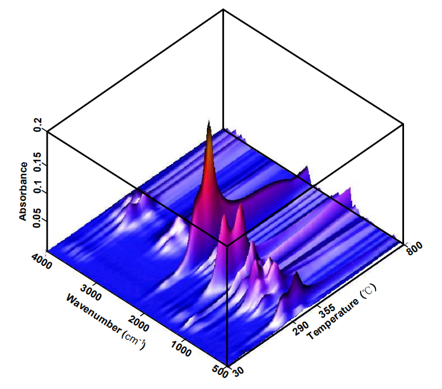Fig. 5. Three-dimensional FTIR image of grape stem at heating rate of 20 °C/min

Based on Fig. 7, the main infrared absorption peaks corresponding to the volatile substances were 2357, 1797, and 1172 cm-1. Some commonly found stretching vibrations of functional groups were easily identified, such as OH stretching vibration at 4000 to 3400 cm-1; CH stretching vibration at 3000 to 2700 cm-1; C=O stretching vibration at 2400 to 2500 cm-1; CO stretching vibration at 2250 to 2000 cm-1; C=O bending vibration at 586 to 750 cm-1; acidic C=O stretching vibration at 1900 to 1650 cm-1; the C-O, C-C, and carbon chain skeleton stretching vibrations at 1475 to 1000 cm-1; the aromatic stretching vibration at 1900 to 1650 cm-1; the CO stretching vibration of phenolic functional group at 1300 to 1200 cm-1; and the CO stretching vibration of alcohol functional group in the range 1200 to 1100 cm-1 (Chen et al. 2014; Ma et al. 2015).

Taking 290 °C as an example, a detailed analysis is shown in Fig. 6. According to the characteristics of the functional groups analyzed above, small gaseous molecules (H2O, CH4, CO2, and CO) were easily identified (Ren et al. 2013). Methane (CH4) came primarily from the decomposition of methoxy, methyl, and methylene groups under high temperatures (Vyazovkin 2001). The CO2 was formed by decarboxylation and cleavage of carbonyl groups (Fu et al. 2012). The breakage of ether bonds and C=O bonds likely formed CO (Vamvuka et al. 2003). A detailed analysis of the composition at 355 °C is shown in Fig. 7.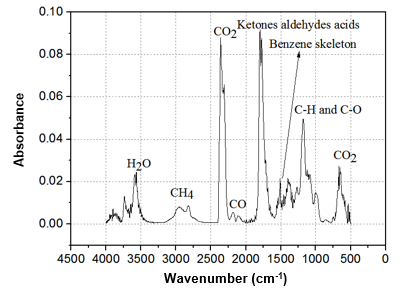Fig. 6. Infrared spectrum of grape stem pyrolytic volatiles at a rate of 20 °C/min and 290 °C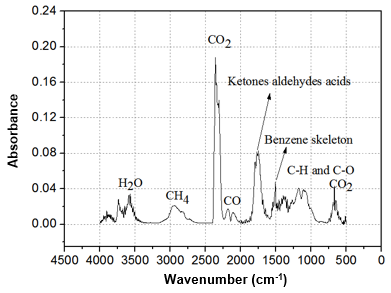Fig. 7. Infrared spectrum of grape stem pyrolytic volatiles with heating rate of 20 °C/min and 355 °C

After identifying the volatile components, the evolution of absorbance intensity of volatile components with increasing temperature was obtained and is shown in Fig. 8. According to Lambert Beer’s law, the absorbance intensity at a specific wave-number is linearly dependent on relative concentration of volatile components (Fu et al. 2012). Therefore, the evolution of absorbance intensity in the whole pyrolysis process represented the tendency of relative concentrations of volatile components. This is in agreement with the DTG curve. Except for CO2, each component displayed two peaks in the whole composition. The first stage (200 °C to 310 °C) involved CO2, aldehydes, ketones, acids, alkanes, alcohols, phenols, ethers, and lipids as the main components, which were mainly derived from hemicellulose pyrolysis. Although in the second stage (310 °C to 400 °C), three types of substances still dominated, which were CO2, CO, and H2O, and aromatic content noticeably increased. This was because a large amount of cellulose and a small part of lignin were pyrolyzed. In the third stage (slow pyrolysis stage), the content of all volatile components gradually decreased, and the upper and lower sides slightly fluctuated.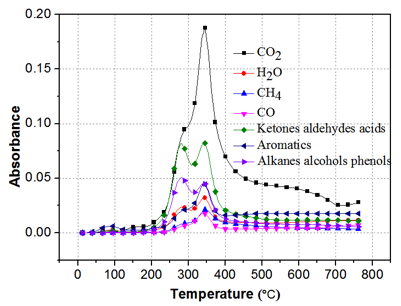Fig. 8. Infrared spectra of grape stem pyrolytic volatiles with the temperature increased at the rate of 20 °C/min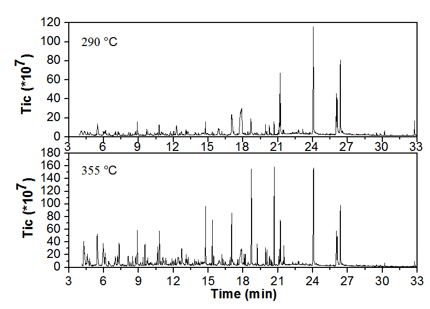Fig. 9. TIC image of grape stem rapid pyrolysis in 290 and 355 °C

Figure 9 shows the total ion chromatogram of the rapid pyrolysis of the grape stem at 290 °C and 355 °C. At a pyrolysis temperature of 355 °C, 3 to approximately 22 min produced a large amount of organic matter as compared with 290 °C. Table 2 indicates statistics at 290 °C grape stem rapid pyrolysis products through the NIST library. The relative content of individual compound peaks in the range of 3.5 min to 33 min was the percentage of the total compound peak area, and the contents of 24 of the products were above 0.6%. While, in rapid pyrolysis products of 355 °C, 35 kinds of products with a relative content above 0.5% were counted, and they are shown in Table 3.

Table 2. Qualitative and Quantitative Analysis of Grape Stem Pyrolysis Products at 290 °C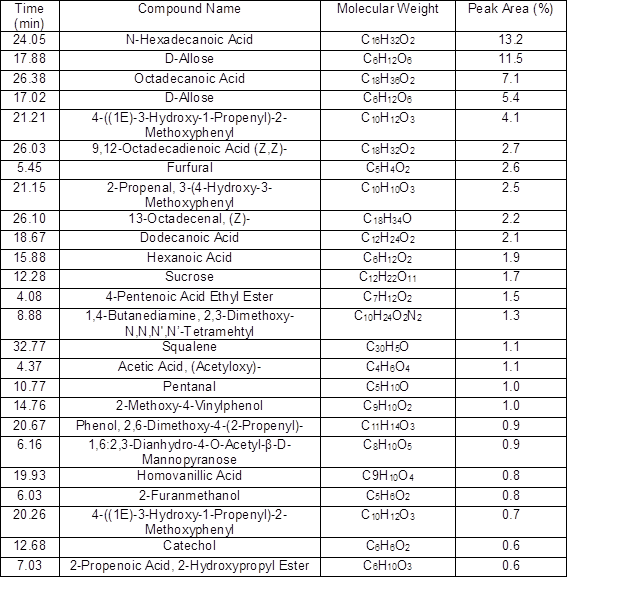From Table 2, it can be seen that the top 5 products were hexadecanoic acid (13.2%), allose (16.9%), stearic acid (7.1%), pine glycosides (4.1%), and linoleic acid (2.7%). It can be observed that the remaining 19 or so substances still indicated a large amount at this temperature. Many macromolecular substances were not cracked, which indicated that the temperature of the grape stem half fiber was decomposed, and cellulose and lignin were only partially decomposed.

Table 3. Qualitative and Quantitative Analysis of Grape Stem Pyrolysis Products in 355 °C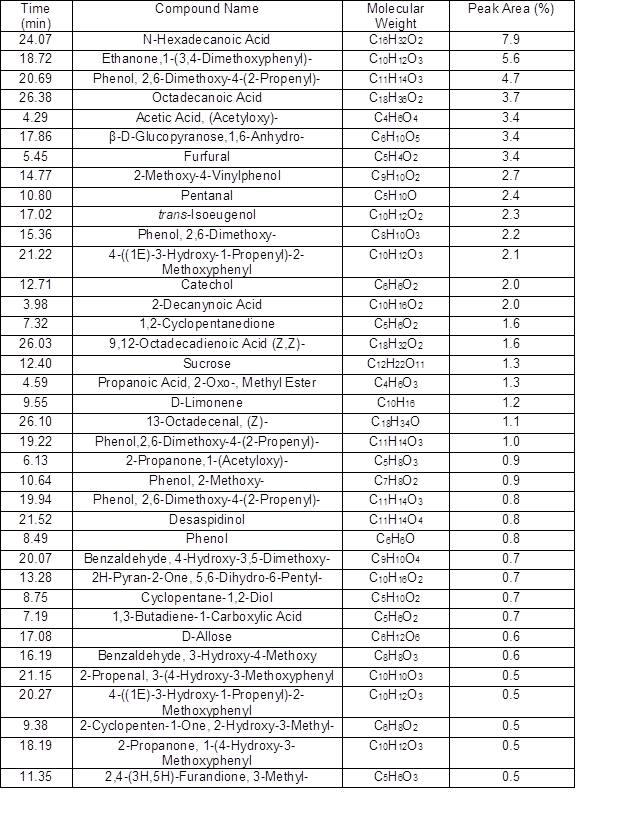Table 3 indicates the pyrolysis products from 355 °C decomposition temperature, of which the top five were ethanone, 1-(3,4-dimethoxyphenyl) [ether 5.6%], hexadecanoic acid (7.9%), phenol, 2,6-dimethoxy-4-(2-propenyl) [phenols 5.7%], stearic acid (3.7%), and acetic acid (3.4%).

In the remaining 30 substances, the relative content of sugar was very low and almost no sugar peaks were visible, indicating that at this temperature, many macromolecular organic components were rapidly cracking, and the grape stem cellulose also underwent rapid decomposition.

Figure 10 is a comparison chart of the main organic products in the rapid pyrolysis of grape stems at 290 °C and 355 °C. It shows that the total relative content of acids at 290 °C reached approximately 31%.

When the cracking temperature was increased to 355 °C, the total content of the acid decreased to approximately 20% and produced only acetic acid. The total relative content of saccharides reached approximately 18% at 290 °C, but at 355 °C, the sugar content was only approximately 2%, almost completely decomposed at 355 °C. The relative content of phenols and aldehydes rapidly increased, while the relative content of phenols increased to approximately 15%, and the aldehyde content increased to approximately 11%.

The relative content of ketones increased to approximately 3% and the contents of ether and aromatic hydrocarbons decreased to nearly zero. This was because at this temperature, the main effect involved the decomposition of cellulose and the formation of aromatic hydrocarbons from the decomposition of lignin. Table 4 lists the main studies in literature related to pyrolysis of biomass.